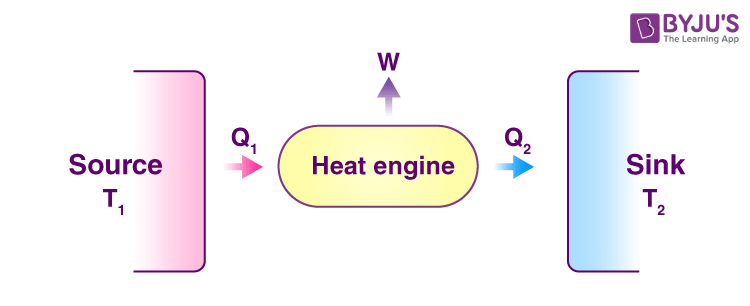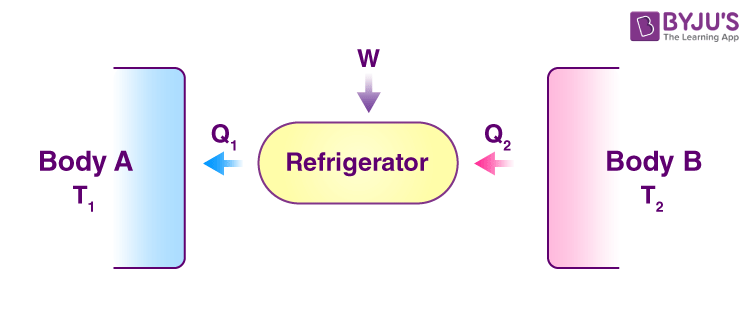# Heat Engine Efficiency

## What is Heat Engine?

A heat engine is a device that converts heat to work. It takes heat from a reservoir, then does some work like moving a piston, lifting weight etc. and finally discharges some heat energy into the sink. Schematically it can be represented as:## Heat Engine Efficiency

Let us derive an expression for the efficiency of a heat engine. We can define heat engine efficiency as:

$$\begin{array}{l}η = W{Q_1}\end{array}$$

Where,

W = Work done by the engine

Q1 = Heat taken from the source

After each cycle, the engine returns to its initial state, so,

$$\begin{array}{l}∆U = 0\end{array}$$

So from the figure, it is clear that,

$$\begin{array}{l}W = Q_1- Q_2\end{array}$$

Hence the heat engine efficiency is:

$$\begin{array}{l}η = \frac{Q_1- Q_2}{Q_1}\end{array}$$

$$\begin{array}{l}η = 1- \frac{Q_2}{Q_1}\end{array}$$

So for Q2 = 0 efficiency will be 100% but, in actual, this is not possible because there will be some loss of energy in the system. Hence, for every engine, there is a limit for its efficiency. The efficiency is maximum for a reversible engine such as Carnot heat engine.## Types of Heat Engine

Following are the two types of heat engines:

• Internal combustion engine
• External combustion engine

### External combustion engine

In these heat engines, the fuel burns outside and away from the main engine, where force and motion are produced. A steam engine is an example of an external combustion engine.

### Internal combustion engine

In these heat engines, the fuel burns inside the cylinder. A car engine is an example of an internal combustion engine.

The internal combustion engine is more efficient than the external combustion engine as there is no energy wasted during heat transfer between the boiler and the cylinder.

Following is the table explaining related concepts of heat engine:

## What is Heat Pump?

A heat pump is a device that extracts heat from one piece and transfers it to another. Heat pumps transfer heat by circulating a substance known as refrigerant through a cycle of evaporation and condensation.

Refrigerators and air conditioners are examples of heat pumps.  A refrigerator is essentially an insulated box with a heat pump system connected to it. The evaporator coil is located inside the box, usually in the freezer compartment. Heat is absorbed from this location and transferred outside, usually behind or underneath the condenser coil’s unit.

A refrigerator takes out heat from a lower temperature T2 and releases it to a higher temperature T1. For this process to occur, work must be done on the system. Below is the schematic representation of the process:In this case, we define the coefficient of performance as:

$$\begin{array}{l}α = \frac{Q_2}{W}\end{array}$$

Where,

• Q2 is the heat taken from the system
• W is the work done on the refrigerator

Similar to heat engines after a cycle, the refrigerator returns to its original state hence ∆U = 0. So from the figure,

$$\begin{array}{l}W = Q_1 – Q_2\end{array}$$

Therefore,

$$\begin{array}{l}α = \frac{Q_2}{Q_1 – Q_2}\end{array}$$

A refrigerator cannot function without external work so its coefficient of performance can never be infinite.

Test your knowledge on Heat engine and its efficiency

1. Nice post

2. Find the efficiency in carnot engine.

• You can read about the efficiency of the Carnot engine here.

3. Very essential and useful for revision at all times

4. What is the difference between a heat engine and a refrigerator?

• In a heat engine, heat is transferred from a higher temperature level (source) to lower temperature level(sink). Work is obtained during this process.

In a refrigerator, the heat is transferred from a lower temperature level to a higher temperature level by applying external work to maintain the temperature below atmospheric temperature.

Both these devices work on the basis of heat flow.